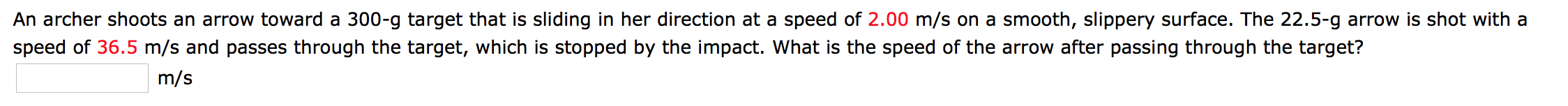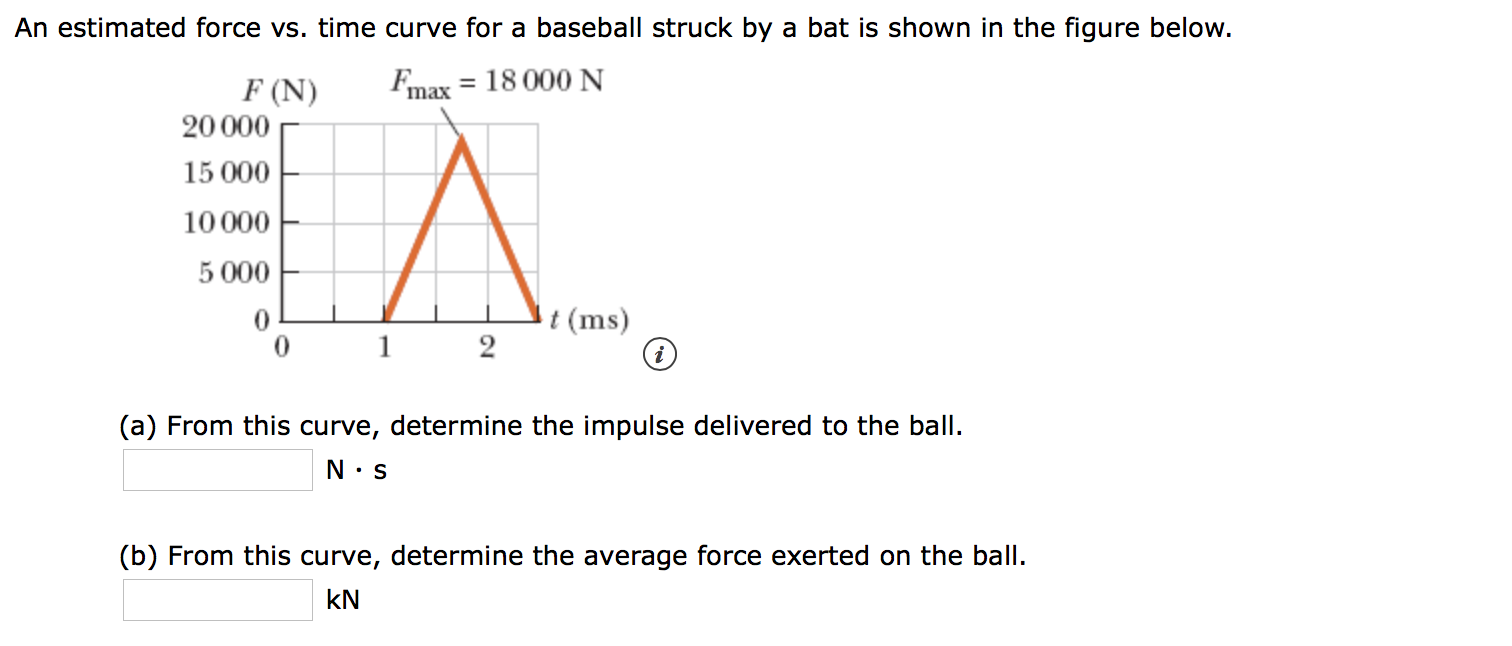# An archer shoots an arrow toward a 300-g target that is sliding in her direction at a speed of 2.00 m/s on a smooth, slippery surface. The 22.5-g arrow is shot with aspeed of 36.5 m/s and passes through the target, which is stopped by the impact. What is the speed of the arrow after passing through the target?m/s An estimated force vs. time curve for a baseball struck by a bat is shown in the figure below.Fmax = 18 000 NF (N)A20 00015 000100005 000t(ms)21(a) From this curve, determine the impulse delivered to the ballN S(b) From this curve, determine the average force exerted on the ballkN

Question
6 viewshelp_outlineImage TranscriptioncloseAn archer shoots an arrow toward a 300-g target that is sliding in her direction at a speed of 2.00 m/s on a smooth, slippery surface. The 22.5-g arrow is shot with a speed of 36.5 m/s and passes through the target, which is stopped by the impact. What is the speed of the arrow after passing through the target? m/s fullscreenhelp_outlineImage TranscriptioncloseAn estimated force vs. time curve for a baseball struck by a bat is shown in the figure below. Fmax = 18 000 N F (N) A 20 000 15 000 10000 5 000 t(ms) 2 1 (a) From this curve, determine the impulse delivered to the ball N S (b) From this curve, determine the average force exerted on the ball kN fullscreen
check_circle

Step 1

Since we only answer the first question, we’ll answer the first one. Please resubmit the question and specify the other question you’d like answered.

Given, subscript 1 is for target and 2 is for arrow i.e.:

Step 2

Using the definition of momentum (p) and law of conservation of linear momentum which states initial momentum (pi) equals final momentum (pf) we get:

Step 3

After the impact the target momentum will be z...

### Want to see the full answer?

See Solution

#### Want to see this answer and more?

Solutions are written by subject experts who are available 24/7. Questions are typically answered within 1 hour.*

See Solution
*Response times may vary by subject and question.
Tagged in

### Physics